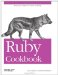# Using Complex Numbers

Problem

You want to represent complex ("imaginary") numbers and perform math on them.

Solution

Use the Complex class, defined in the complex library. All mathematical and trigonometric operations are supported.

```	require 'complex'

Complex::I # => Complex(0, 1)

a = Complex(1, 4) # => Complex(1, 4)
a.real # => 1
a.image # => 4

b = Complex(1.5, 4.25) # => Complex(1.5, 4.25)
b + 1.5 # => Complex(3.0, 4.25)
b + 1.5*Complex::I # => Complex(1.5, 5.75)

a - b # => Complex(-0.5, -0.25)
a * b # => Complex(-15.5, 10.25)
b.conjugate # => Complex(1.5, -4.25)
Math::sin(b) # => Complex(34.9720129257216, 2.47902583958724)
```

Discussion

You can use two floating-point numbers to keep track of the real and complex parts of a complex number, but that makes it complicated to do mathematical operations such as multiplication. If you were to write functions to do these operations, you'd have more or less reimplemented the Complex class. Complex simply keeps two instances of Numeric, and implements the basic math operations on them, keeping them together as a complex number. It also implements the complex-specific mathematical operation Complex#conjugate.

Complex numbers have many uses in scientific applications, but probably their coolest application is in drawing certain kinds of fractals. Here's a class that uses complex numbers to calculate and draw a character-based representation of the Mandelbrot set, scaled to whatever size your screen can handle.

```	class Mandelbrot

# Set up the Mandelbrot generator with the basic parameters for
# deciding whether or not a point is in the set.

def initialize(bailout=10, iterations=100)
@bailout, @iterations = bailout, iterations
end
```

A point (x,y) on the complex plane is in the Mandelbrot set unless a certain iterative calculation tends to infinity. We can't calculate "tends towards infinity" exactly, but we can iterate the calculation a certain number of times waiting for the result to exceed some "bail-out" value.

If the result ever exceeds the bail-out value, Mandelbrot assumes the calculation goes all the way to infinity, which takes it out of the Mandelbrot set. Otherwise, the iteration will run through without exceeding the bail-out value. If that happens, Mandelbrot makes the opposite assumption: the calculation for that point will never go to infinity, which puts it in the Mandelbrot set.

The default values for bailout and iterations are precise enough for small, chunky ASCII renderings. If you want to make big posters of the Mandelbrot set, you should increase these numbers.

Next, let's define a method that uses bailout and iterations to guess whether a specific point on the complex plane belongs to the Mandelbrot set. The variable x is a position on the real axis of the complex plane, and y is a position on the imaginary axis.

```	# Performs the Mandelbrot operation @iterations times. If the
# result exceeds @bailout, assume this point goes to infinity and
# is not in the set. Otherwise, assume it is in the set.
def mandelbrot(x, y)
c = Complex(x, y)
z = 0
@iterations.times do |i|
z = z**2 + c # This is the Mandelbrot operation.
return false if z > @bailout
end
return true
end
```

The most interesting part of the Mandelbrot set lives between2 and 1 on the real axis of the complex plane, and between1 and 1 on the complex axis. The final method in Mandelbrot produces an ASCII map of that portion of the complex plane. It maps each point on an ASCII grid to a point on or near the Mandelbrot set. If Mandelbrot estimates that point to be in the Mandelbrot set, it puts an asterisk in that part of the grid. Otherwise, it puts a space there. The larger the grid, the more points are sampled and the more precise the map.

```	def render(x_size=80, y_size=24, inside_set="*", outside_set=" ")
0.upto(y_size) do |y|
0.upto(x_size) do |x|
scaled_x = -2 + (3 * x / x_size.to_f)
scaled_y = 1 + (-2 * y / y_size.to_f)
print mandelbrot(scaled_x, scaled_y) ? inside_set : outside_set
end
puts
end
end
end
```

Even at very small scales, the distinctive shape of the Mandelbrot set is visible.

```	Mandelbrot.new.render(25, 10)
# **
# ****
# ********
# *** *********
# *******************
# *** *********
# ********
# ****
# **
```

• The scaling equation, used to map the complex plane onto the terminal screen, is similar to the equations used to scale data in Recipe 12.5, "Adding Graphical Context with Sparklines," and Recipe 12.14, "Representing Data as MIDI Music"Ruby Cookbook (Cookbooks (OReilly))
ISBN: 0596523696
EAN: 2147483647
Year: N/A
Pages: 399